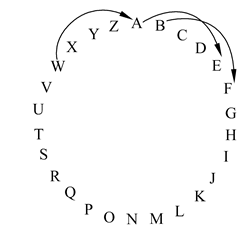# C++程序设计实例

【例3.12】用下面公式求π的近似值。π/4≈1-1/3+1/5-1/7+…直到最后一项的绝对值小于10-7为止。根据给定的算法很容易编写程序如下：

1. #include <iostream>
2. #include <iomanip>
3. #include <cmath>
4. using namespace std;
5. int main( )
6. {
7. int s=1;
8. double n=1,t=1,pi=0;
9. while((fabs(t))>1e-7)
10. {
11. pi=pi+t;
12. n=n+2;
13. s=-s;
14. t=s/n;
15. }
16. pi=pi*4;
17. cout<<"pi="<<setiosflags(ios::fixed)<<setprecision(6)<<pi<<endl;
18. return 0;
19. }

pi=3.141592

【例3.13】求Fibonacci数列前40个数。这个数列有如下特点：第1、2个数为1、1。从第3个数开始，每个数是其前面两个数之和。即：
F1=1  (n=1)
F2=1  (n=2)
Fn=Fn-1+Fn-2(n≥3)

1. #include <iostream>
2. #include <iomanip>
3. using namespace std;
4. int main( )
5. {
6. long f1,f2;
7. int i;
8. f1=f2=1;
9. for(i=1;i<=20;i++)
10. {
11. cout<<setw(12)<<f1<<setw(12)<<f2;
12. //设备输出字段宽度为12,每次输出两个数
13. if(i%2==0) cout<<endl;
14. //每输出完4个数后换行,使每行输出4个数
15. f1=f1+f2;
16. //左边的f1代表第3个数,是第1､2个数之和
17. f2=f2+f1;
18. //左边的f2代表第4个数,是第2､3个数之和
19. }
20. return 0;
21. }

【例3.14】找出100~200间的全部素数。编写程序如下：

1. #include <iostream>
2. #include <cmath>
3. #include <iomanip>
4. using namespace std;
5. int main( )
6. {
7. int m,k,i,n=0;
8. bool prime;//定义布尔变量prime
9. for(m=101;m<=200;m=m+2) //判别m是否为素数,m由101变化到200,增量为2
10. {
11. prime=true;//循环开始时设prime为真,即先认为m为素数
12. k=int(sqrt(m)); //用k代表根号m的整数部分
13. for(i=2;i<=k;i++) //此循环作用是将m被2~根号m除,检查是否能整除
14. if(m%i==0) //如果能整除,表示m不是素数
15. {
16. prime=false; //使prime变为假
17. break; //终止执行本循环
18. }
19. if (prime)//如果m为素数
20. {
21. cout<<setw(5)<<m; //输出素数m,字段宽度为5
22. n=n+1; //n用来累计输出素数的个数
23. }
24. if(n%10==0) cout<<endl; //输出10个数后换行
25. }
26. cout<<endl;//最后执行一次换行
27. return 0;
28. }

【例3.15】译密码。1. #include <iostream>
2. using namespace std;
3. int main( )
4. {
5. char c;
6. while ((c=getchar( ))!='\n')
7. {
8. if((c>='a' && c<='z') || (c>='A' && c<='Z'))
9. {
10. c=c+4;
11. if(c>'Z' && c<='Z'+4 || c>'z')
12. c=c-26;
13. }
14. cout<<c;
15. }
16. cout<<endl;
17. return 0;
18. }

I am going to Beijing!↙
M eq ksmrk xs Fimnmrk!

while语句中括号内的表达式有3个作用：
• 从键盘读入一个字符，这是用getchar函数实现的；
• 将读入的字符赋给字符变量c；
• 判别这个字符是否为'\n'(即换行符)。如果是换行符就执行while语句中的复合语句(即花括号内的语句)，对输入的非换行符的字符进行转换处理。

if (c>'Z'|| c>'z')  c=c-26;

(0)
0%

(0)
0%